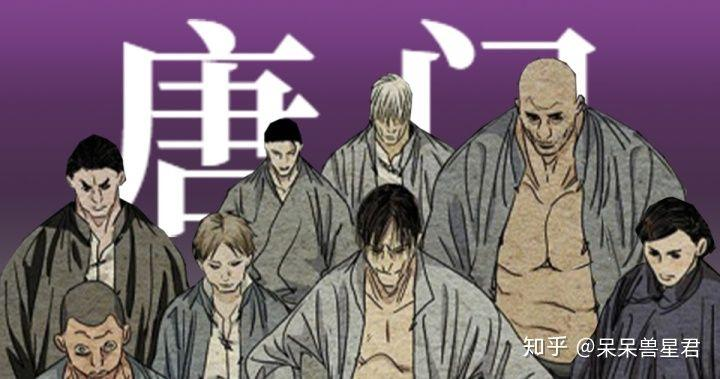（杜佛嵩；唐同壁等人介于二三世代之间）；

（陶桃；马龙等人介于四五世代之间）；

20年八月；腾讯漫画官方突然在一人之下的漫画评论区提出了一个问题：如果唐门有八奇技，会是什么样的？

1.炁毒

``一代``
• 唐家仁

``二代``
• 高英才

``二代半``
• 杜佛嵩

• 唐同壁

``三代``
• 许新

• 董昌

``四代半``
• 高少山

``五代``
• 未知

2.幻身障

``一代``
• 唐家仁

``二代``
• 高英才

``三代``
• 许新

• 杨烈

• 唐妙兴

3.观

``二代``
• 唐明夷

``四代半``
• 胖子

``五代``
• 未知

4.瞬击

``一代``
• 唐家仁

``二代半``
• 唐同壁；杜佛嵩

``三代``
• 唐妙兴

``四代半``
• 园儿

5.机关

``二代``
• 王离

``二代半``
• 李鼎

``三代``

• 使用配合观园的追踪器
``四代半``
• 韩寅

``五代``
• 高楠

6.五宝护身法

• 土木流注

``四代半``
• 马龙

• 园儿

• 陶桃

7.毒障

``一代``
• 唐家仁

``二代``
• 唐明夷

``三代``
• 董昌

• 唐秋山

``四代半``
• 陶桃

• 未知

• 未知

``五代``
• 唐文龙

• 柳飞熊

8.丹噬

``一代``
• 唐家仁

``三代``
• 杨烈

• 许新

• 唐妙兴

1.唐门御物

``二代``
• 高英才

``五代``
• 未知（疑似）

• 未知

2.唐门暗器

``一代``
• 唐家仁（手刺）

``二代``
• 王离（手刺）

• 高英才（隐线）

``二代半``
• 杜佛嵩（手刺）

``三代``
• 杨烈（隐线）

• 许新（隐线）

• 许新（球形暗器）

• 唐秋山（针）

``五代``
• 唐婷婷（隐线）

• 未知（球形暗器）

• 唐文龙（暗器）

3.絮步

``四代半``
• 园儿